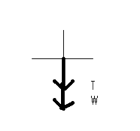# Examples

A 1.5 kg bucket of water is tied by a rope and whirled in a circle with a radius of 1.0 m. At the top of the circular loop, the speed of the bucket is 4.0 m/s. Determine the acceleration, the net force and the individual force values when the bucket is at the top of the circular loop.

A free body diagram for this situation would be as follows:Tension is the mechanical force transmitted to the string and it is directed toward the center of the circle and Weight is the force due to gravity which is always downward.

Notice that centripetal force is NOT placed on the free body diagram since it is the force that is due to the sum of the tension in the string and the weight.

The centripetal force, Fc, is directed downward though, and it is important to know that. For this example, then, the tension and the weight are both in the same direction as the centripetal force. First, we can find the centripetal acceleration, ac, since ac = v2/r.

ac = v2/r

ac = (4 m/s)2/1 m = 16 m/s2

Then , T + W = Fc

T + mg = mac

T = mac – mg

T = (1.5 kg)(16 m/s2) – (1.5 kg)(10 m/s2)

T = 9 N

The net force on the bucket when it is at the top of the circle is then the sum of the weight and tension and since both are directed downward, they would have negative values. Therefore the net force on the bucket is -9N + (-15 N) = -24 N

Example #2:

A 1.5 kg bucket of water is tied by a rope and whirled in a circle with a radius of 1.0 m. At the bottom of the circular loop, the speed of the bucket is 6.0 m/s. Determine the acceleration, the net force and the individual forces when the bucket is at the bottom of the circular loop.

Similar to the first problem, but now the bucket is at the bottom of the loop, so the forces will point in somewhat different directions. A free body diagram of this situation would be as follows:This time, the tension points upward toward the center of the circle and the weight is always down so they are in opposite directions. The centripetal force is always directed towards the center of the circle (up or positive in this case). Solving for the centripetal acceleration first as above,

ac = v2/r = (6 m/s)2/1.0 m = 36 m/s2

T – W = +Fc

T = Fc + W

T = mac + mg

T = (1.5 kg)(36 m/s2) + (1.5 kg)(10 m/s2) = 69 N

The net force is the sum of the forces of tension and weight and they are now in opposite directions with the tension positive and weight negative. Therefore, 69 N – 15 N = 54 N.

(source)